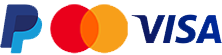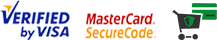# Chargeable weight rules

## What is Chargeable Weight (Volumetric Weight)?

 The volumetric weight of a shipment is a calculation that reflects the density of a package. A less dense item generally occupies more volume of space, in comparison to its actual weight. The volumetric or dimensional weight is calculated and compared with the actual weight of the shipment to ascertain which is greater; the higher weight is used to calculate the shipment cost.### Calculation

• Multiply the length x height x width of your parcel in centimetres.
• Divide the answer by 5,000.
• The result is the volumetric weight. This figure should be compared with the actual weight in kilogrammes. Whichever is the greater figure will be used to calculate your consignment price.
• Please note that you need to round all weights up to the next half kilogram.

#### For example

Let's say the actual weight of your parcel is 10 kg, and dimensions are 30cm x 50cm x 50cm.
• 30cm x 50cm x 50cm = 75000
• 75000 / 5000 = 15kg

The volumetric weight is therefore 15kg, which is greater than the actual weight of 10kg, and so the volumetric weight would be used in calculating the price to be charged.

Please follow this link to find out maximum dimensions per parcel.

•